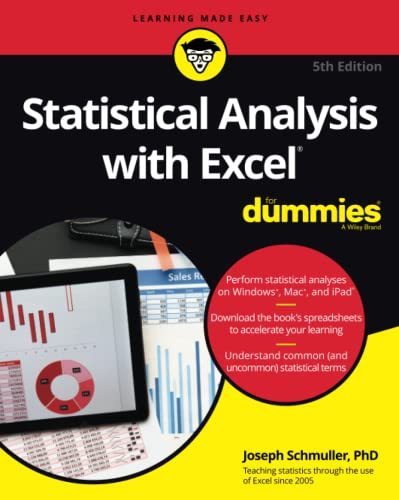# Statistical Analysis with Excel For Dummies, 5th Edition

Rating: 3.8 out of 5 129 reviews
• Length: 576 pages
• Edition: 5
• Publisher:
• Publication Date: 2021-12-30
• ISBN-10: 1119844541
• ISBN-13: 9781119844549

## Book Description

Become a stats superstar by using Excel to reveal the powerful secrets of statistics

Microsoft Excel offers numerous possibilities for statistical analysis—and you don’t have to be a math wizard to unlock them. In Statistical Analysis with Excel For Dummies, fully updated for the 2021 version of Excel, you’ll hit the ground running with straightforward techniques and practical guidance to unlock the power of statistics in Excel.

Bypass unnecessary jargon and skip right to mastering formulas, functions, charts, probabilities, distributions, and correlations. Written for professionals and students without a background in statistics or math, you’ll learn to create, interpret, and translate statistics—and have fun doing it!

In this book you’ll find out how to:

• Understand, describe, and summarize any kind of data, from sports stats to sales figures
• Confidently draw conclusions from your analyses, make accurate predictions, and calculate correlations
• Model the probabilities of future outcomes based on past data
• Perform statistical analysis on any platform: Windows, Mac, or iPad
• Access additional resources and practice templates through Dummies.com

For anyone who’s ever wanted to unleash the full potential of statistical analysis in Excel—and impress your colleagues or classmates along the way—Statistical Analysis with Excel For Dummies walks you through the foundational concepts of analyzing statistics and the step-by-step methods you use to apply them.

``````Part 1 Getting Started with Statistical Analysis with Excel: A Marriage Made in Heaven
Chapter 1 Evaluating Data in the Real World
Chapter 2 Understanding Excel’s Statistical Capabilities

Part 2 Describing Data
Chapter 3 Show-and-Tell: Graphing Data
Chapter 5 Deviating from the Average
Chapter 6 Meeting Standards and Standings
Chapter 7 Summarizing It All
Chapter 8 What’s Normal?

Part 3 Drawing Conclusions from Data
Chapter 9 The Confidence Game: Estimation
Chapter 10 One-Sample Hypothesis Testing
Chapter 11 Two-Sample Hypothesis Testing
Chapter 12 Testing More Than Two Samples
Chapter 13 Slightly More Complicated Testing
Chapter 14 Regression: Linear and Multiple
Chapter 15 Correlation: The Rise and Fall of Relationships
Chapter 17 Nonparametric Statistics

Part 4 Probability
Chapter 18 Introducing Probability
Chapter 19 More on Probability
Chapter 20 Using Probability: Modeling and Simulation
Chapter 21 Estimating Probability: Logistic Regression

Part 5 The Part of Tens
Chapter 22 Ten (12, Actually) Statistical and Graphical Tips and Traps
Chapter 23 Ten Topics (Thirteen, Actually) That Just Don’t Fit Elsewhere

Part 6 Appendices
Appendix A When Your Data Live Elsewhere
Appendix B Tips for Teachers (and Learners)
Appendix C More on Excel Graphics
Appendix D The Analysis of Covariance``````